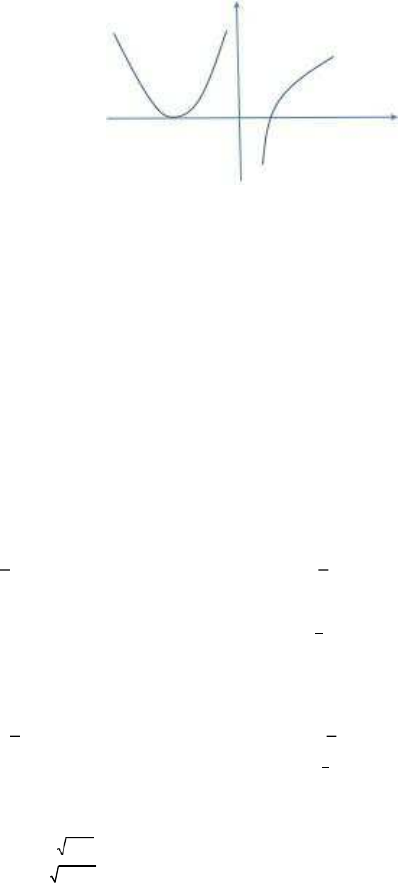• # 2015年考研数学三真题及答案

杰克陈

3

0

3.0分

共90页 2021-10-25 5研币
1
一、选择题1-8 小题，每小 4 分，共 32 分．
1.
x
n
是数列，下列命题中不正确的是
(A)
lim
x
n
a
lim
x
2n
lim
x
2n
1
a
n

n

n

(B)
lim
x
2n
lim
x
2n
1
a
lim
x
n
a
n

n

n

(C)
lim
x
n
a
lim
x
3n
lim
x
3n
1
a
n

n

n

(D)
lim
x
3n
lim
x
3n
1
a
lim
x
n
a
n

答案
(
D
)
n

n

解析
】这个题目考查的是收敛的数列与其子数列间的关系，如果数列
{
x
n
}
收敛于
a
，那么它的
一子数列也收敛，且极限也a
(
A
)
选项， lim
x
n
=
a
也是
{
x
n
}
收敛于
a
那么
{
x
2n
}
,
{
x
2n
+
1
}
{
x
n
}
n
lim
x
2n
lim
x
2n
1
a ，选项
(
A
)
是对的。对于选项
(
C
)
(
A
)
是一样的道理。所以选项
n

n

(
C
)
也是正确的
(
B
)
选项，
{
x
2n
}
,
{
x
2n
+
1
}
的极限都是等于
a
那么
{
x
2n
}
,
{
x
2n
+
1
}
分为下标为偶数和奇数
lim
x
n
=
a 以选
(
B
)
是对的
n
(
D
)
选项，
lim
x
3n
lim
x
3n
1
a
{
x
3n
}
,
{
x
3n
+
1
}
{
x
n
}
子列，所以不能推
lim
x
n
a
n

所以
(
D
)
选项错。
n

n

2.
f
x
,
2 阶导函数 f
x
的图形如右图所示，则曲线 y f
x
的拐
2015
年考研数学三真题及答案x
1
2
1
1
x
点个数为（
(A)
)0
答案
(
C
)
(B) 1
(C) 2
(D) 3
解析】这道题目考查的对拐点的断，题目给出 f
(
x
)
的图形，根据判断拐点的第一充
两遍号，。所形上
两边然有以选
(
C
)
3. D
x, y
| x
2
y
2
2x, x
2
y
2
2 y
函数 f x, y D

f x, y dxdy （）
D
(
A
)
4
d
2cos
f
r cos
, r sin
rdr
2
d
2sin
f
r cos
, r sin
rdr
0
0
2sin
0
4
2cos
(
B
)
4
d
f
r cos
, r sin
rdr
2
d
f
r cos
, r sin
rdr
0
0
0
4
(
C
)2
0
dx f
x, y
dy
1 2x
x
2
(
D
)2
0
dx
x
f
x, y
dy
答案
(
B
)
立即下载
评论(0)
没有更多评论了哦～

## 热门标签

• 官方
微信• 微信
客服• QQ
客服